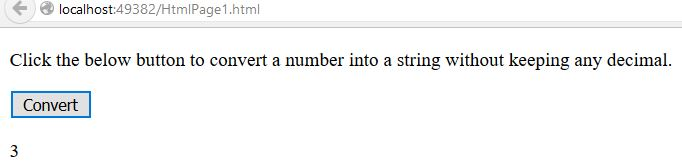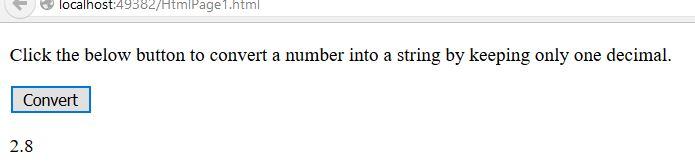# ToFixed() Method in JavaScript

##### How to convert a number into string without keeping any decimals in JavaScript?

By using `toFixed()` method we can convert a number into string without keeping any decimals.

### CASE 1: Converting a number into string without keeping any decimals.

```<p>Click the below button to convert a number into a string without keeping any decimal.</p>
<input type="button" value="Convert" onclick="Function()" />
<p id="myId"></p>

<script>
function Function() {
var A = 2.74562;
var R = A.toFixed();
document.getElementById("myId").innerHTML = R;
}
</script>
```

In the above code snippet we have given `Id` as "`myId`"to the second `<p>` element in the HTML code. There is a Function() in the`<script>`block which is connected to the Onclick of the HTML button. We need to convert a number into string without keeping any decimals, for that we are using `toFixed()`, this method gives the approximate nearer value of the decimal. The `document.getElementByI``d("myId") ` returns the element that has Id "id=myID" in the HTML page. Onclick of the button "Convert" fires the Function() in the <script> block at the same time `toFixed()` method convert a number into string and gives the output as normal value without having any decimals.

OUTPUT### CASE 2: Convert a number into string, keeping only one decimal.

```<p>Click the below button to convert a number into a string by keeping only one decimal.</p>
<input type="button" value="Convert" onclick="Function()" />
<p id="myId"></p>

<script>
function Function() {
var A = 2.79562;
var R = A.toFixed(1)
document.getElementById("myId").innerHTML = R;
}
</script>
```

toFixed()

In the above code snippet we used `toFixed(1)`in the `<script>` block which convert the number into string and gives the output as number with only one decimal.

OUTPUTNOTE: By increasing and decresing the value of `toFixed()` method we can reduce and increase the decimals.

Views: 3378 | Post Order: 90